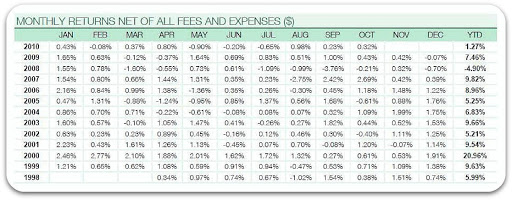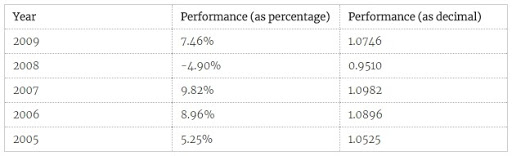### Understanding Compound and Annualised Growth

Across fund manager houses, there is little standardisation of how performance figures are presented on fact sheets. Percentage returns are usually presented as either (i) discrete (or calendar) annual, (ii) cumulative (for example a total return figure for 3 years or 5 years) or (iii) annualised (the equivalent annualised return for a period such as 3 or 5 years, sometimes called compound annual return).

This tutorial shows you how to convert from discrete annual performance figures, to cumulative or annualised figures, over a period of your choice.

As an example, we’ll look at some USD fund performance data presented in an October 2010 factsheet.The performance data are presented to us in a very clear way, but may not be the complete picture we are looking for. The table directly above shows the discrete annual returns - ie. net of fees the fund delivered 7.46% in 2009, -4.90% in 2008, 9.82% in 2007, etc.

But let’s suppose you ask yourself (or your advisor) “What was the total growth for the five years 2005-2009, and what is that as an annual rate?” How do we calculate those figures?

If we had the actual unit price data, the job is easier. But with performance data given as percentages we can still arrive at the answer with reasonable accuracy, using the geometic mean method.

## Finding The Cumulative Return

We don’t find cumulative return simply by adding each year’s percentage figure. We must multiply each year’s figure together, expressed as a decimal multiplier. To do this, we divide by 100 and add 1. So, 7.46% becomes 1.0746 (i.e. 1+0.0746), -4.90% becomes 0.9510 (i.e. 1-0.0490), etc.Multiplying each figure in the right column (1.0746 x 0.9510 x 1.0982 x …) gives us 1.287057. We convert back to percentage exactly the reverse of above: subtract 1 and multiply by 100 (rounding up if desired).

So, 1.287057 is the same as 28.70% total growth (cumulative return) over the five year period. Not too bad for the period concerned, but would a bank deposit account have been better? To know that, we need the annualised return.

## Finding The Annualised Return

The equation for annualised return i is

$$i = [ (1 + r)^{1/n} - 1 ] \times 100$$
(otherwise known as the compound annual growth rate formula CAGR); where r is the total cumulative return over the whole period of n years.

In our example,
$$i = [ (1+0.2870)^{1/5} - 1 ] \times 100 = 5.176\% \space p.a.$$

In other words, over the selected five year period the return delivered has been equivalent to saving in a deposit account at a fixed rate of 5.176% per annum.

You can double-check this with the total growth formula:

$$(1 + 0.05176)^5 = 1.2870 \space \space i.e. 28.70\%$$
(which is where we started!).